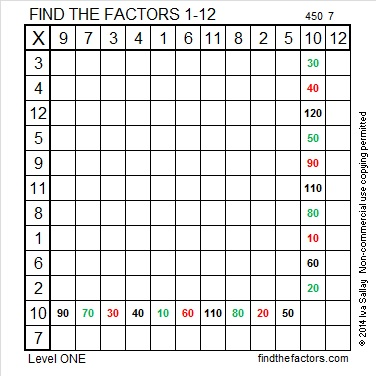# 323 and Level 1

• 323 is a composite number.
• Prime factorization: 323 = 17 x 19
• The exponents in the prime factorization are 1 and 1. Adding one to each and multiplying we get (1 + 1)(1 + 1) = 2 x 2 = 4. Therefore 323 has exactly 4 factors.
• Factors of 323: 1, 17, 19, 323
• Factor pairs: 323 = 1 x 323 or 17 x 19
• 323 has no square factors that allow its square root to be simplified. √323 ≈ 17.972Since 17 × 19 = 323, we know that 323 + 1 = 324 = 18². That follows from the fact that (n – 1)(n + 1) = n² – 1 is ALWAYS true.

Because 17 is one of its factors, 323 is the hypotenuse of a Pythagorean triple:

• 152-285-323 which is 19 times 8-15-17

Here are 20 very easy clues to help you solve this puzzle:Print the puzzles or type the factors on this excel file: 12 Factors 2014-12-15